# Positive-definite form

Jump to: navigation, search

An expressionwhere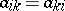, which takes non-negative values for any real valuesand vanishes only for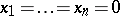. Therefore, a positive-definite form is a quadratic form of special type. Any positive-definite form can be converted by a linear transformation to the representation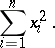In order that a form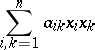be positive definite, it is necessary and sufficient that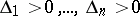, where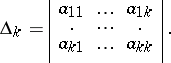In any affine coordinate system, the distance of a point from the origin is expressed by a positive-definite form in the coordinates of the point.

A form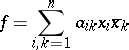such that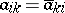andfor all values ofandonly foris called a Hermitian positive-definite form.

The following concepts are related to the concept of a positive-definite form: 1) a positive-definite matrix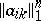is a matrix such that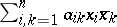is a Hermitian positive-definite form; 2) a positive-definite kernel is a function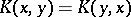such thatfor every function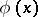with an integrable square; 3) a positive-definite function is a functionsuch that the kernelis positive definite. By Bochner's theorem, the class of continuous positive-definite functionswith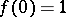coincides with the class of characteristic functions of distributions of random variables (cf. Characteristic function).

#### Comments

A kernel that is semi-positive definite (non-negative definite) is one that satisfies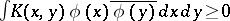for all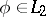. Such a kernel is sometimes also simply called positive. However, the phrase "positive kernel" is also used for the weaker notion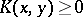(almost-everywhere). A positive kernel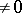in the latter sense has at least one eigen value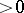while a semi-positive definite kernel has all eigen values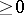.